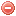# Description

The Fire weather index ($$FWI$$) is the final index of the $$FWI$$ system. It consists of the combination of the $$ISI$$ and $$BUI$$ and is a measure of fire intensity in the form of energy output rate per unit length of fire front. Nevertheless, the $$FWI$$ is appropriated for predicting various aspects of fire activity as it combines all influencing factors in one number (Van Wagner 1987).

# Formula

The previous version of the $$FWI$$ was measured on scale from 0 to 16 (the D-scale). For different reasons, this D-scale was judged inappropriate and a new scale was developed: the I-scale. As its values were judged too high, the I-scale was substituted by a reduced function (square root) of itself, the B-scale. However, the B-scale still not being optimal, a new scale was finally developed: the S-scale. For further details about the development of the different $$FWI$$ scales, see Van Wagner (1987).

The equations for converting the S-scale into the I-scale when fire intensity is expressed in kilowatts per meter are the following (Van Wagner 1987):

$\ln S=1.013\cdot{\{\ln {(0.289\cdot I)}\}}^{0.647}$

$\ln {(0.289\cdot I)}=0.980\cdot{(\ln S)}^{1.546}$

The $$FWI$$ is a measure of fire intensity and thus requires for its computation factors representing rate of spread and fuel consumption. While the $$ISI$$ represents rate of spread, this is not the case for the $$BUI$$. In order to convert the $$BUI$$ into a measure of weight of fuel consumed, $$f(D)$$, the following equation is needed (Van Wagner 1987):

$f(D) = \begin{cases} 0.626\cdot{BUI^{0.809}}+2, & \mbox{for }BUI\leqslant{80} \\ & \\ \dfrac{1000}{25+108.64\cdot{e^{-0.023\cdot{BUI}}}}, & \mbox{for }BUI>{80} \end{cases}$

where $$BUI$$ is the Buildup index.

Then, the B-scale $$FWI$$ is obtained as follows:

$B=0.1\cdot{ISI}\cdot{f(D)}$

And the S-scale $$FWI$$ as follows:

$S = \begin{cases} e^{2.72\cdot{(0.434\cdot\ln B)}^{0.647}}, & \mbox{for }B>{1} \\ & \\ B, & \mbox{for }B\leqslant{1} \end{cases}$

# Interpretation of the index

While the $$FWI$$ scale is uniform everywhere, this is not the case for the range of fire weather. Therefore, it is required to define fire danger classes (e.g. very low, low, moderate, etc.) within the $$FWI$$ scale fitting regional or local fire weather patterns. A procedure in order to develop a rational class breakdown (and an example) is proposed in Van Wagner (1987):
"First, compile a historical sample of the $$FWI$$ over a number of seasons. Second, decide how many Extreme days should be allowed each season on the average, and set the lower limit of the Extreme class. Third, arrange the other classes on a geometric progression in terms of the I-scale, using a constant ratio of I-scale value from class to class. Finally, convert the I-scale values back to the S-scale $$FWI$$. Alternately, the $$FWI$$ scale may simply be divided to provide some predetermined proportion of days in each class."

Original publication:
Van Wagner (1987)

### Bibliography SearchNo results!

No results were found. Are you sure you searched for a tag?

### Symbols

 Variable Description Unit $$T$$ air temperature °C $$T_{dew}$$ dew point temperature °C $$H$$ air humidity % $$P$$ rainfall mm $$U$$ windspeed m/s $$w$$ days since last rain (or rain above threshold) d $$rr$$ days with consecutive rain d $$\Delta t$$ time increment d $$\Delta{e}$$ vapor pressure deficit kPa $$e_s$$ saturation vapor pressure kPa $$e_a$$ actual vapor pressure kPa $$p_{atm}$$ atmospheric pressure kPa $$PET$$ potential evapotranspiration mm/d $$r$$ soil water reserve mm $$r_s$$ surface water reserve mm $$EMC$$ equilibrium moisture content % $$DF$$ drought factor - $$N$$ daylight hours hr $$D$$ weighted 24-hr average moisture condition hr $$\omega$$ sunset hour angle rad $$\delta$$ solar declination rad $$\varphi$$ latitude rad $$Cc$$ cloud cover Okta $$J$$ day of the year (1..365/366) - $$I$$ heat index - $$R_n$$ net radiation MJ⋅m-2⋅d-1 $$R_a$$ daily extraterrestrial radiation MJ⋅m-2⋅d-1 $$R_s$$ solar radiation MJ⋅m-2⋅d-1 $$R_{so}$$ clear-sky solar radiation MJ⋅m-2⋅d-1 $$R_{ns}$$ net shortwave radiation MJ⋅m-2⋅d-1 $$R_{nl}$$ net longwave radiation MJ⋅m-2⋅d-1 $$\lambda$$ latent heat of vaporization MJ/kg $$z$$ elevation m a.s.l. $$d_r$$ inverse relative distance Earth-Sun - $$\alpha$$ albedo or canopy reflection coefficient - $$\Delta$$ slope of the saturation vapor pressure curve kPa/°C $$Cc$$ cloud cover eights $$ROS$$ rate of spread m/h $$RSF$$ rate of spread factor - $$WF$$ wind factor - $$WRF$$ water reserve factor - $$FH$$ false relative humidity - $$FAF$$ fuel availability factor - $$PC$$ phenological coefficient -

 Suffix Description $$-$$ mean / daily value $$_{max}$$ maximum value $$_{min}$$ minimum value $$_{12}$$ value at 12:00 $$_{13}$$ value at 13:00 $$_{15}$$ value at 15:00 $$_{m}$$ montly value $$_{y}$$ yearly value $$_{f/a}$$ value at fuel-atmosphere interface $$_{dur}$$ duration $$_{soil}$$ value at soil level

 Constant Description $$e$$ Euler's number $$\gamma$$ psychrometric constant $$G_{SC}$$ solar constant $$\sigma$$ Stefan-Bolzmann constant